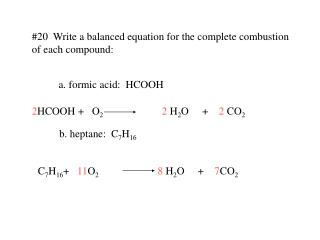DownloadDownload Presentation#20 Write a balanced equation for the complete combustion of each compound:

# #20 Write a balanced equation for the complete combustion of each compound:

Télécharger la présentation## #20 Write a balanced equation for the complete combustion of each compound:

- - - - - - - - - - - - - - - - - - - - - - - - - - - E N D - - - - - - - - - - - - - - - - - - - - - - - - - - -
##### Presentation Transcript

1. #20 Write a balanced equation for the complete combustion of each compound: a. formic acid: HCOOH 2HCOOH + O22 H2O + 2 CO2 b. heptane: C7H16 C7H16+ 11O28 H2O + 7CO2

2. 21. Write a balanced equation for the complete combustion of glucose: C6H12O6 C6H12O6+ O2 H2O + CO2 6 6 6

3. DECOMPOSITION

4. SINGLE-REPLACEMENT

5. DOUBLE-REPLACEMENT

6. COMBUSTION

7. #23 What are the keys to predicting the products of the five general types of reactions? • combination: Two elements combine to form one new • compound. • decomposition: one compound breaks up into its elements. • single replacement: one element replaces another in a • compound. • 4. double replacement: two compounds exchange ions to form • two new compounds. • 5. combustion: a fuel reacts with oxygen gas to form carbon • dioxide and water vapor.

8. #24 Classify each reaction and balance the equations: a. C3H6 + O2 CO2 + H2O 2 9 3 6 3 6 combustion b. Al(OH)3 Al2O3 + H2O 2 3 decomposition c. Li + O2 Li2O 4 2 combination d. Zn + AgNO3 Ag + Zn(NO3)2 2 2 single replacement

9. #25 Which of the five general types of reactions would most likely occur, given each set of reactants? What are the probable products? • an aqueous solution of two ionic compouds double replacement is most likely. The most likely products are two new compounds. b. a single compound a decomposition is most likely. The elements from which this compound is composed are the likely products. c. two elements a combination is most likely. The likely product will be a “combination” of the two elements d. oxygen and a compound of carbon and hydrogen combustion. The likely products are CO2 and H2O

10. #26 complete and balance an equation for each reaction: a. CaI2 + Hg(NO3)2 ? (HgI2 precipiates) a. CaI2 + Hg(NO3)2 HgI2(s) + Ca(NO3)2 b. Al + Cl2 ? b. 2Al + 3Cl2 2AlCl3 c. Ag + HCl  ? c. 2Ag + 2HCl  2AgCl + H2(g) d. C2H2 + O2 ? d. 2C2H2 + 5O2 4CO2 + 2H2O e. MgCl2  ? e. MgCl2  Mg + Cl2

11. #27 What are the three types of products that result in double-replacement reactions? A precipitate gas Water